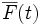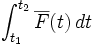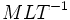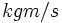# Impulse

## Definition

The impulse due to a force applied over a period of time is the integral of the force over the period of time. In other words, the impulse due to a force$\overline{F}(t)$ (a vector quantity) from time$t = t_1$ to time$t = t_2$ is given by$\int_{t_1}^{t_2} \overline{F}(t) \, dt$

Impulse is responsible for a change in the linear momentum, and the net impulse on a system equals, as a vector, the change in the linear momentum of the system. This is one of the many formulations of Newton's second law.

The concept of impulse is particularly useful for collisions, where a very large and quickly changing quantity of force is exerted by the two bodies on each other over a very short interval of time. Measuring the force as a function of time is hard, but the total value of the impulse can both be measured and theoretically predicted. In the study of collisions, the impulse is typically further broken down into normal impulse and frictional impulse.

## Units and dimensions

MLT dimensions$MLT^{-1}$ (type MLT;1;1;-1)
SI units$kgm/s$ (kilogram meters per second) or$Ns$ (Newton-second)